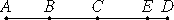# Proposition 23

If as many odd numbers as we please are added together, and their multitude is odd, then the sum is also odd.

Let as many odd numbers as we please, AB, BC, and CD, the multitude of which is odd, be added together.

I say that the sum AD is also odd.(VII.Def.7)

Subtract the unit DE from CD, therefore the remainder CE is even.

But CA is also even, therefore the sum AE is also even.

(VII.Def.7)

And DE is a unit. Therefore AD is odd.

Therefore, if as many odd numbers as we please are added together, and their multitude is odd, then the sum is also odd.

Q.E.D.

## Guide

Like the previous proposition, the proof of this one relies on an unproved statement in VII.Def.7.

This proposition is used in propositions IX.29 and IX.30.